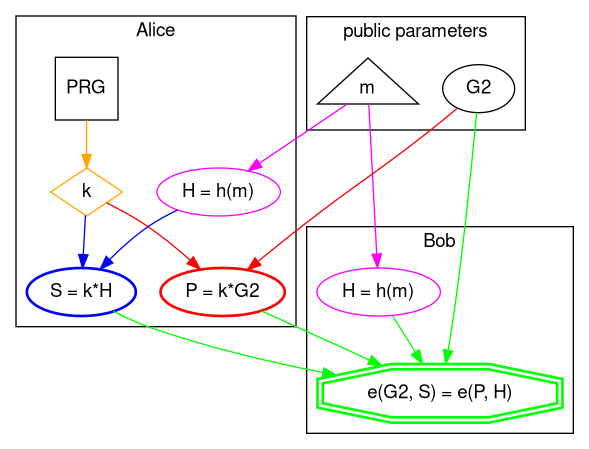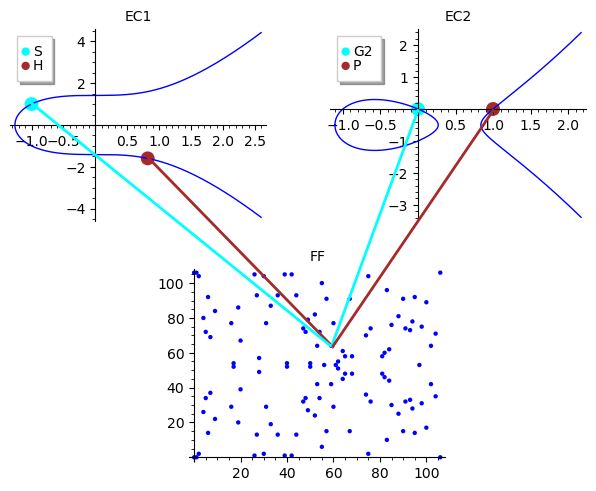# BLS signature scheme

## Cryptography

From a high level perspective this is how BLS (also known as Boneh–Lynn–Shacham) signature scheme works.import sys
sys.path.append("/home/icostan/Repos/pairings.py" )
import bn256

# helper function
def h(msg: bytes) -> object:
return bn256.g1_hash_to_point(msg);

# public parameters
m = b"Hello BLS!"
G2 = bn256.twist_G

# Alice
k = bn256.rand_elem() # private key (orange)
P = G2.scalar_mul(k)  # public key generation (red)
H = h(m)              # hash to curve point (magenta)
S = H.scalar_mul(k)   # signing (blue)
# Alice sends public key 'P' and signature 'S' to Bob

# Bob
H = h(m)                        # hash to curve point (magenta)
v1 = bn256.optimal_ate(G2, S)   # signature pairing
v2 = bn256.optimal_ate(P, H)    # message pairing
"YOU ARE A CRYPTOSTAR!" if v1 == v2 else "YOU SUCK!" # verification (green)
'YOU ARE A CRYPTOSTAR!'


### Nomenclature

A bit of naming conventions first, because shapes, colors, and names have meaning:

#### Names

• capital letter: point on elliptic curve
• lower case: integer or bytes array
• *: elliptic curve (scalar) multiplication
• PRG: Pseudo-Random Generator

#### Shapes

• ellipse: elliptic curve operation, points on curves
• rectangle: number generator
• diamond: large integer (scalar)
• double octagon: pairings
• triangle: bytes array

#### Colors

• orange: private key
• magenta: hash the message to elliptic curve point
• red: public key generation
• blue: signing
• green: verification

### Details

#### 0. Library

Import BN256 elliptic curve pairing library.

  import sys
sys.path.append("/home/icostan/Repos/pairings.py" )
import bn256
bn256
<module 'bn256' from '/home/icostan/Repos/pairings.py/bn256.py'>


#### 1. Public parameters

Besides message m and generator G2 that are shown below we also know:

• elliptic curves EC2 and EC1 with generator G1
• target extension field TF.

but they are hidden in Barreto-Naehrig 256-bit (BN256) curve implementation.

  # helper function
def h(msg: bytes) -> object:
return bn256.g1_hash_to_point(msg);

# public parameters
m = b"Hello BLS!"
G2 = bn256.twist_G
type(G2)
<class 'bn256.curve_twist'>


#### 2. Key generation

This is as simple as randomly generating private key k then multiply by generator G2 to obtain the public key, which is another point on EC2.

  # Alice
k = bn256.rand_elem() # private key (orange)
P = G2.scalar_mul(k)  # public key generation (red)
type(P)
<class 'bn256.curve_twist'>


#### 3. Signing

To sign the message m Alice has to hash the message to elliptic curve and get a point on EC1 then multiply that by private key k to obtain the signature S which is a point on EC1.

  H = h(m)              # hash to curve point (magenta)
S = H.scalar_mul(k)   # signing (blue)
type(S)
# Alice sends public key 'P' and signature 'S' to Bob
<class 'bn256.curve_point'>


#### 4. Verification

Bob receives public key P and signature S from Alice and hashes the message to curve to calculate the same H point in EC1.

Now comes the beautiful, yet so powerful part of pairing-based cryptography. Bob uses generator G2 and signature S to calculate one side of the equation, then public key P and point H to calculate the other side, if equality holds then signature is valid.

  # Bob
H = h(m)                        # hash to curve point (magenta)
v1 = bn256.optimal_ate(G2, S)   # signature pairing
v2 = bn256.optimal_ate(P, H)    # message pairing
type(v2)
"YOU ARE A CRYPTOSTAR!" if v1 == v2 else "YOU SUCK!" # verification (green)
<class 'bn256.gfp_12'>
'YOU ARE A CRYPTOSTAR!'


#### Pairing intuition

Now, the math behind pairings is quite complicated and to be honest I do not fully understand it (yet) but at least we can have a simplified visual intuition using 2 elliptic curves over rational numbers and a finite field.You can also check the references below, lots of good resources to learn from.

Happy pairing!